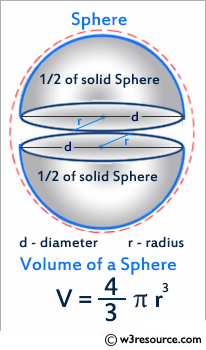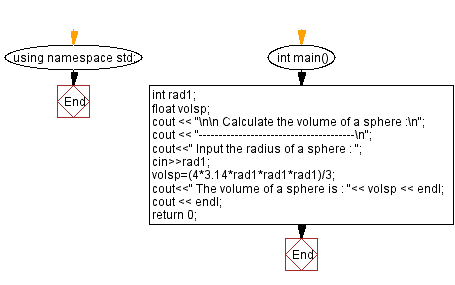﻿ C++ Exercises: Calculate the volume of a sphere - w3resource

# C++ Exercises: Calculate the volume of a sphere

## C++ Basic: Exercise-14 with Solution

Write a C++ program that calculates the volume of a sphere.

Pictorial Presentation:Sample Solution:

C++ Code :

``````#include <iostream> // Including the input-output stream header file

using namespace std; // Using the standard namespace

int main() // Start of the main function
{
float volsp; // Declaring a floating-point variable volsp to store the volume of the sphere
cout << "\n\n Calculate the volume of a sphere :\n"; // Outputting a message indicating the calculation of sphere volume
cout << "---------------------------------------\n"; // Outputting a separator line

cout << " Input the radius of a sphere : "; // Prompting the user to input the radius of the sphere
cin >> rad1; // Taking input for the radius from the user

// Calculating the volume of the sphere using the formula: (4/3) * π * r^3

cout << " The volume of a sphere is : "<< volsp << endl; // Displaying the calculated volume of the sphere
cout << endl; // Outputting a blank line for better readability

return 0; // Returning 0 to indicate successful program execution
} // End of the main function
``````

Sample Output:

``` Calculate the volume of a sphere :
---------------------------------------
Input the radius of a sphere : 6
The volume of a sphere is : 904.32
```

Flowchart:C++ Code Editor:

What is the difficulty level of this exercise?

﻿